#### Bandwidth Restriction

Because the areal segments that are generated from the angle and distance classes increase in area as the lag distance increases, it is sometimes desirable to restrict this area (Deutsch and Journel, 1992, p. 45). If you specify the BANDWIDTH= option in the COMPUTE statement, the lateral, or perpendicular, distance from the axis that defines the angle classes is fixed.

For example, suppose two points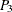,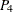are picked from the domain in Figure 102.19 and are superimposed on the grid that defines distance and angle classes, as shown in Figure 102.23.

The endpoint of vector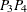falls within the angle class around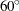and the fifth lag class; however, it falls outside the restricted area that is defined by the bandwidth. Hence, it is excluded from the semivariance calculation.

Figure 102.23: Selected PairFalls outside Bandwidth Limit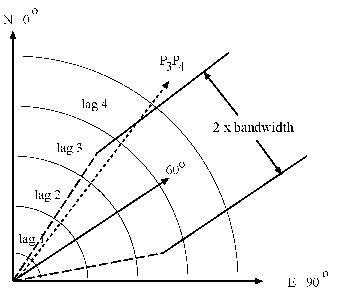Finally, a pair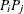that falls in a lag class larger than the value of the MAXLAGS= option is excluded from the semivariance calculation.

The BANDWIDTH= option complements the angle and lag tolerances in determining how point pairs are included in distance classes. Clearly, the number of pairs within each angle/distance class is strongly affected by the angle and lag tolerances and whether BANDWIDTH= has been specified. See also the section Angle Classification for more details about the effects these rules can have, since BANDWIDTH= operates in a manner similar to the ANGLETOLERANCE= option.